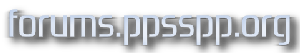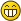Custom PPSSPP shaders - Printable Version +- forums.PPSSPP.org (https://forums.ppsspp.org) +-- Forum: PPSSPP - Playstation Portable Simulator Suitable for Playing Portably (/forumdisplay.php?fid=1) +--- Forum: Development (/forumdisplay.php?fid=3) +--- Thread: Custom PPSSPP shaders (/showthread.php?tid=6594) RE: Custom PPSSPP shaders - LunaMoo - 11-14-2015 06:08 PM Heh reuploaded again, @t3nsini sorry for that - should be fixed now. I think my gpu's drivers are auto correcting common mistakes/typos or something since that was caused by something I missed when converting one filter from HLSL to GLSL. Suprising that your nvidia gpu doesn't do that, it's neither bad nor old and should be more friendly for mistakes than my amd gpu's.(Edit: I would guess that might be due to windows 10 which drivers certainly aren't as good nor polished as on other systems yet.) Either way I hope nothing more like that is hidding there, unfortunately since it works for me due to some magic, can't be certain of that anymore. RE: Custom PPSSPP shaders - t3nsini - 11-15-2015 11:50 PM LunaMoo Worked!!! YAY!!! Thx! U´re the best!RE: Custom PPSSPP shaders - Nick001 - 11-21-2015 03:38 PM (11-07-2015 04:16 AM)[Unknown] Wrote:   (11-07-2015 12:13 AM)LunaMoo Wrote:  Yeah about games pausing rendering for loading screens and such we probably can't do much at least not in a way it currently works. That sounds like something we could add a flag to the post shader ini to change. Not volunteering to implement it, but it ought to be doable. -[Unknown] ALL the cookies to whoever would do that. Seriously all of them. Though I do realize it would take time away from more important issues. RE: Custom PPSSPP shaders - dj001 - 11-25-2015 01:07 PM Hi. This is possible to disable Smooth Icons. Like item icons, sword icon near hero name... I can't disable this. RE: Custom PPSSPP shaders - shinra358 - 11-28-2015 12:31 PM Can you add the spline shader and port/replace byuu's scanlines from Higan (his is easier to configure) OR add an image overlay shader (displays image from a png and adjusts transparency)? GLSL Documentation / Tutorial - Cel Shading Wind Waker - eddiefur - 12-21-2015 05:07 PM Hello everyone, I started working on a shader that looks like the cel shading used in Zelda Wind Waker. I'm only limited to the actual codes of the shaders available with ppsspp. Those shader are kind of hard to understand because I'm new with the GLSL and those shaders use non meaningful variables and there is no comments... I would like to know ALL variables I can used from PPSSPP to make the best shader as possible. I mean, can I have access to vertex normal, lighting or any sort of matrix? The actual shader from ppsspp use only textures and texel (I don't know what it is). A lot of websites tell that cel shading is based on normal and lighting... and that's where I'm stucked... I tried to do my cel shading wind waker like with only textures informations and I've got some results but not as good as I expected... Can you tell me where I can find some documentation to help me with my shader creation? Thank you, sorry for my language, I'm french... RE: GLSL Documentation / Tutorial - Cel Shading Wind Waker - eddiefur - 12-22-2015 05:22 PM I think I've got some great results so far... there is the code : Vertex Shader : attribute vec4 a_position; attribute vec2 a_texcoord0; varying vec4 v_texcoord0; void main() { gl_Position = a_position; v_texcoord0=a_texcoord0.xyxy; } Fragment shader : uniform sampler2D sampler0; varying vec4 v_texcoord0; vec3 rgb2hsv(vec3 c) { vec4 K = vec4(0.0, -1.0 / 3.0, 2.0 / 3.0, -1.0); vec4 p = mix(vec4(c.bg, K.wz), vec4(c.gb, K.xy), step(c.b, c.g)); vec4 q = mix(vec4(p.xyw, c.r), vec4(c.r, p.yzx), step(p.x, c.r)); float d = q.x - min(q.w, q.y); float e = 1.0e-10; return vec3(abs(q.z + (q.w - q.y) / (6.0 * d + e)), d / (q.x + e), q.x); } vec3 hsv2rgb(vec3 c) { vec4 K = vec4(1.0, 2.0 / 3.0, 1.0 / 3.0, 3.0); vec3 p = abs(fract(c.xxx + K.xyz) * 6.0 - K.www); return c.z * mix(K.xxx, clamp(p - K.xxx, 0.0, 1.0), c.y); } void main() { vec3 ct = texture2D(sampler0, v_texcoord0.zw).xyz; vec3 hsv = rgb2hsv(ct); if (hsv.z >= 0 && hsv.z < 0.05) hsv.z = 0.025; else if (hsv.z >= 0.05 && hsv.z < 0.2) hsv.z = 0.125; else if (hsv.z >= 0.2 && hsv.z < 0.25) hsv.z = 0.225; else if (hsv.z >= 0.25 && hsv.z < 0.30) hsv.z = 0.275; else if (hsv.z >= 0.30 && hsv.z < 0.45) hsv.z = 0.375; else if (hsv.z >= 0.45 && hsv.z < 0.50) hsv.z = 0.475; else if (hsv.z >= 0.5 && hsv.z < 0.55) hsv.z = 0.525; else if (hsv.z >= 0.55 && hsv.z < 0.70) hsv.z = 0.625; else if (hsv.z >= 0.70 && hsv.z < 0.75) hsv.z = 0.725; else if (hsv.z >= 0.75 && hsv.z < 0.8) hsv.z = 0.775; else if (hsv.z >= 0.8 && hsv.z < 0.95) hsv.z = 0.875; else if (hsv.z >= 0.95 && hsv.z <= 1) hsv.z = 0.975; ct = hsv2rgb(hsv); gl_FragColor.xyz = ct; gl_FragColor.a = 1.0; } RE: GLSL Documentation / Tutorial - Cel Shading Wind Waker - eddiefur - 12-22-2015 08:51 PM fragment shader update : uniform sampler2D sampler0; varying vec4 v_texcoord0; void main() { vec3 ct = texture2D(sampler0, v_texcoord0.zw).xyz; float br = max(max(ct.r, ct.g), ct.b); float nbr; if (br >= 0 && br < 0.05) nbr = 0.025; else if (br >= 0.05 && br < 0.2) nbr = 0.125; else if (br >= 0.2 && br < 0.25) nbr = 0.225; else if (br >= 0.25 && br < 0.30) nbr = 0.275; else if (br >= 0.30 && br < 0.45) nbr = 0.375; else if (br >= 0.45 && br < 0.50) nbr = 0.475; else if (br >= 0.5 && br < 0.55) nbr = 0.525; else if (br >= 0.55 && br < 0.70) nbr = 0.625; else if (br >= 0.70 && br < 0.75) nbr = 0.725; else if (br >= 0.75 && br < 0.8) nbr = 0.775; else if (br >= 0.8 && br < 0.95) nbr = 0.875; else if (br >= 0.95 && br <= 1) nbr = 0.975; float mul = nbr / br; ct *= mul; gl_FragColor.xyz = ct; gl_FragColor.a = 1.0; } My new shader - Nintendo DS Auto Contrast like - eddiefur - 01-03-2016 12:08 AM Hello everyone, here's a shader I came with today. It's a shader inspired by subtle constrast and contouring we can see in some Nintendo DS games. It adds so much clarity in your psp game. Have fun playing gamesFragment Shader File Content uniform sampler2D sampler0; uniform vec2 u_texelDelta; varying vec4 v_texcoord0; void main() { vec3 cu = texture2D(sampler0, v_texcoord0.xy + (vec2(0.0, -1.0) * u_texelDelta)).xyz; vec3 cd = texture2D(sampler0, v_texcoord0.xy + (vec2(0.0, 1.0) * u_texelDelta)).xyz; vec3 cl = texture2D(sampler0, v_texcoord0.xy + (vec2(-1.0, 0.0) * u_texelDelta)).xyz; vec3 cr = texture2D(sampler0, v_texcoord0.xy + (vec2(1.0, 0.0) * u_texelDelta)).xyz; vec3 c = texture2D(sampler0, v_texcoord0.xy).xyz; float lc = max(max(c.r, c.g), c.b); float d=0; float nd=0; nd = max(max(cu.r-c.r, cu.g-c.g), cu.b-c.b); if(nd > d) d=nd; nd = max(max(cd.r-c.r, cd.g-c.g), cd.b-c.b); if(nd > d) d=nd; nd = max(max(cl.r-c.r, cl.g-c.g), cl.b-c.b); if(nd > d) d=nd; nd = max(max(cr.r-c.r, cr.g-c.g), cr.b-c.b); if(nd > d) d=nd; gl_FragColor.xyz = c * (lc - d) / lc; gl_FragColor.a = 1.0; } Vertex Shader File Content attribute vec4 a_position; attribute vec2 a_texcoord0; varying vec4 v_texcoord0; void main() { gl_Position = a_position; v_texcoord0=a_texcoord0.xyxy; } RE: Custom PPSSPP shaders - vnctdj - 01-03-2016 09:26 AM Merged. RE: Custom PPSSPP shaders - eddiefur - 01-04-2016 03:59 PM Very good shader from http://www.raywenderlich.com/10862/how-to-create-cool-effects-with-custom-shaders-in-opengl-es-2-0-and-cocos2d-2-x. It a MUST HAVE in PPSSPP. It adds so much depth into the games. Fragment Shader Code uniform sampler2D sampler0; uniform vec2 u_texelDelta; varying vec4 v_texcoord0; void main() { vec3 ct = texture2D(sampler0, v_texcoord0.xy).xyz; ct += texture2D(sampler0, v_texcoord0.xy - (vec2(1.0, 1.0) * u_texelDelta)).xyz; ct -= texture2D(sampler0, v_texcoord0.xy + (vec2(1.0, 1.0) * u_texelDelta)).xyz; gl_FragColor.xyz = ct; gl_FragColor.a = 1.0; } Vertex Shader Code attribute vec4 a_position; attribute vec2 a_texcoord0; varying vec4 v_texcoord0; void main() { gl_Position = a_position; v_texcoord0=a_texcoord0.xyxy; } RE: Custom PPSSPP shaders - Demetrius - 01-08-2016 04:12 PM LunaMoo, please check out your PM. Thank youRE: Custom PPSSPP shaders - eddiefur - 01-08-2016 10:36 PM Modification of the emboss / bump map shader. Really much better. Fragment shader file content uniform sampler2D sampler0; uniform vec2 u_texelDelta; varying vec4 v_texcoord0; vec3 emboss(vec2 textcoord, float str) { bool ok = true; vec3 c = texture2D(sampler0, textcoord.xy).xyz; vec3 cp = texture2D(sampler0, textcoord.xy - (vec2(1.0, 1.0) * u_texelDelta)).xyz; vec3 cm = texture2D(sampler0, textcoord.xy + (vec2(1.0, 1.0) * u_texelDelta)).xyz; float l = max(max(c.r, c.g), c.b); float nl; while(ok && str > 0) { nl = l; nl += max(max(cp.r, cp.g), cp.b) * str; nl -= max(max(cm.r, cm.g), cm.b) * str; if(abs(nl - l) < 0.1) ok = false; else str-=0.1; } if(str > 0) return c * nl / l; else return vec3(1, 0, 0); } void main () { gl_FragColor.rgb = emboss(v_texcoord0.xy, 2); // 2 is the strength of the effect... the higher the more strength gl_FragColor.a = 1; } RE: Custom PPSSPP shaders - GamerzHell9137 - 01-09-2016 08:37 AM (01-08-2016 10:36 PM)eddiefur Wrote:  Modification of the emboss / bump map shader. Really much better. Fragment shader file content uniform sampler2D sampler0; uniform vec2 u_texelDelta; varying vec4 v_texcoord0; vec3 emboss(vec2 textcoord, float str) { bool ok = true; vec3 c = texture2D(sampler0, textcoord.xy).xyz; vec3 cp = texture2D(sampler0, textcoord.xy - (vec2(1.0, 1.0) * u_texelDelta)).xyz; vec3 cm = texture2D(sampler0, textcoord.xy + (vec2(1.0, 1.0) * u_texelDelta)).xyz; float l = max(max(c.r, c.g), c.b); float nl; while(ok && str > 0) { nl = l; nl += max(max(cp.r, cp.g), cp.b) * str; nl -= max(max(cm.r, cm.g), cm.b) * str; if(abs(nl - l) < 0.1) ok = false; else str-=0.1; } if(str > 0) return c * nl / l; else return vec3(1, 0, 0); } void main () { gl_FragColor.rgb = emboss(v_texcoord0.xy, 2); // 2 is the strength of the effect... the higher the more strength gl_FragColor.a = 1; } I doubt that anyone understands what you're talking about. If you made a new custom shader then post the file so we can use it in PPSSPP. RE: Custom PPSSPP shaders - eddiefur - 01-10-2016 03:31 PM Yeah but that forum is so limited... we can't upload any pictures or files on the forum... just tell me where to upload the file.